# LeetCode周赛295，赛后看了大佬的代码，受益匪浅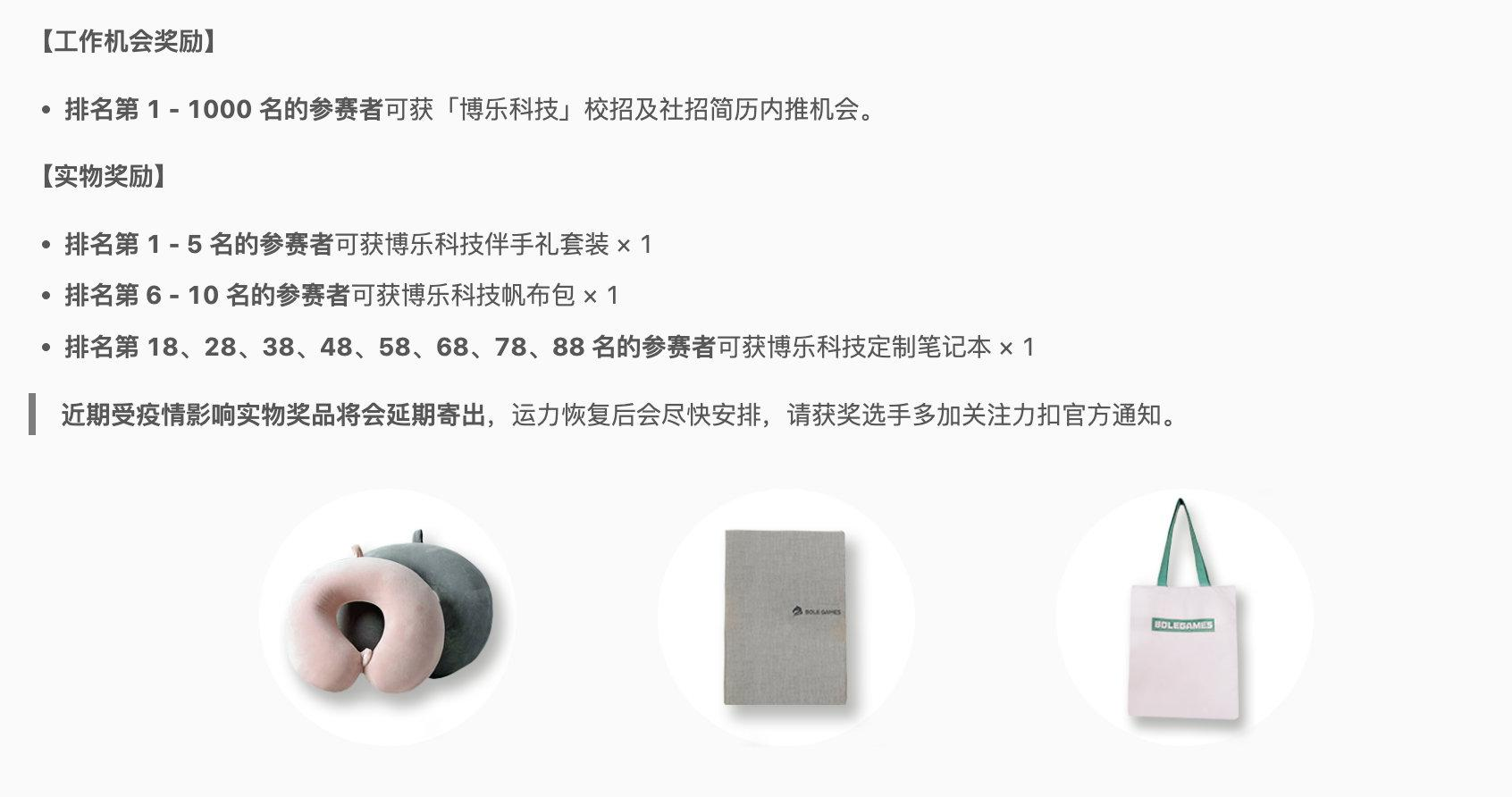## 重排字符形成目标字符串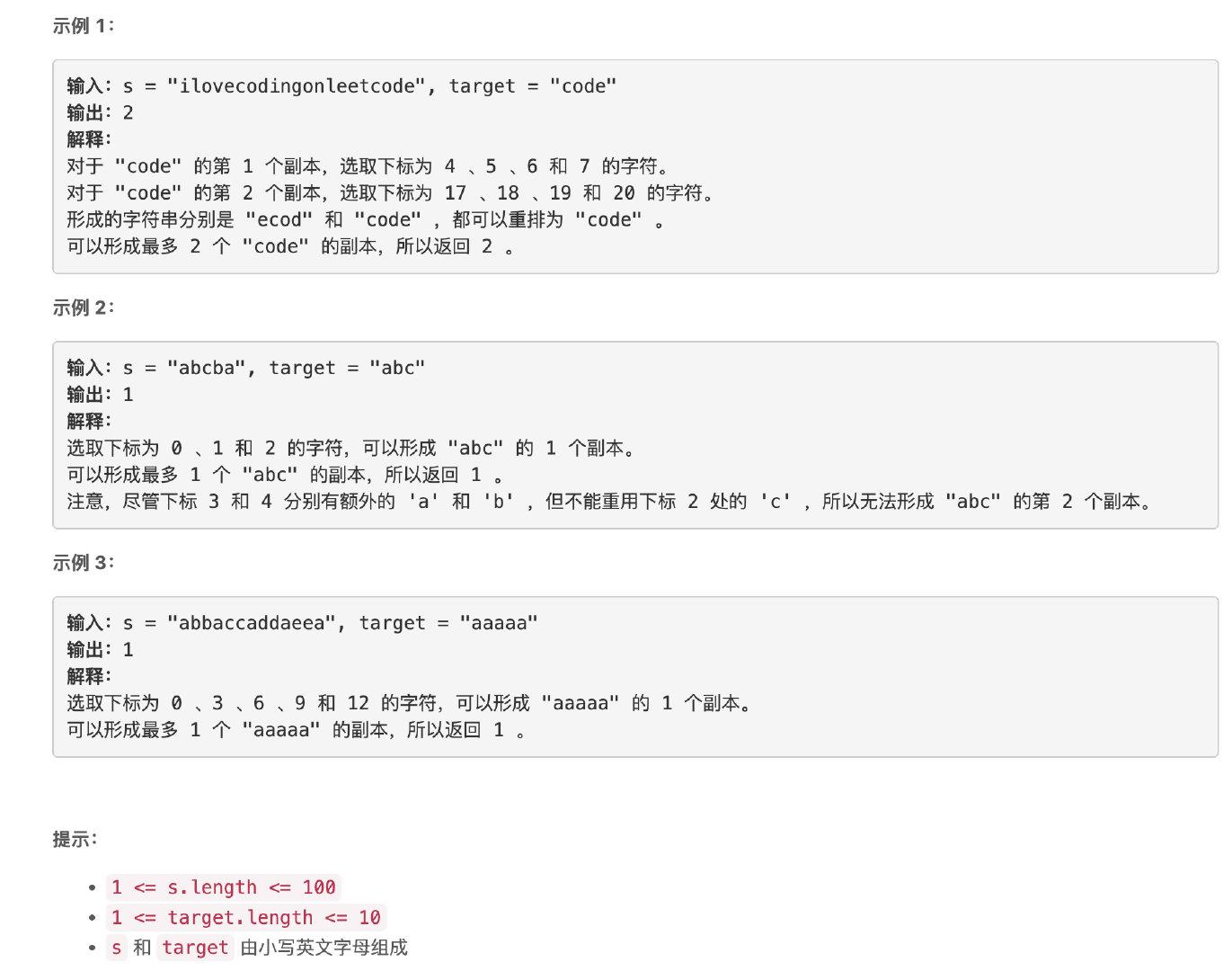### 题解

```cpp class Solution {ub public: int rearrangeCharacters(string s, string target) { map mp, used; int ret = 0; for (auto c : s) { mp[c]++; }

``````    for (auto c : target) {
used[c] ++;
}

ret = 0x3f3f3f3f;

for (auto p = used.begin(); p != used.end(); p++) {
char c = p->first;
int v = p->second;
if (mp.count(c) == 0) return 0;
ret = min(mp[c] / v, ret);
}
return ret;
}
``````

}; ```

## 价格减免

• 例如 "\$100"、"\$23" 和 "\$6.75" 表示价格，而 "100"、"\$" 和 "2\$3" 不是。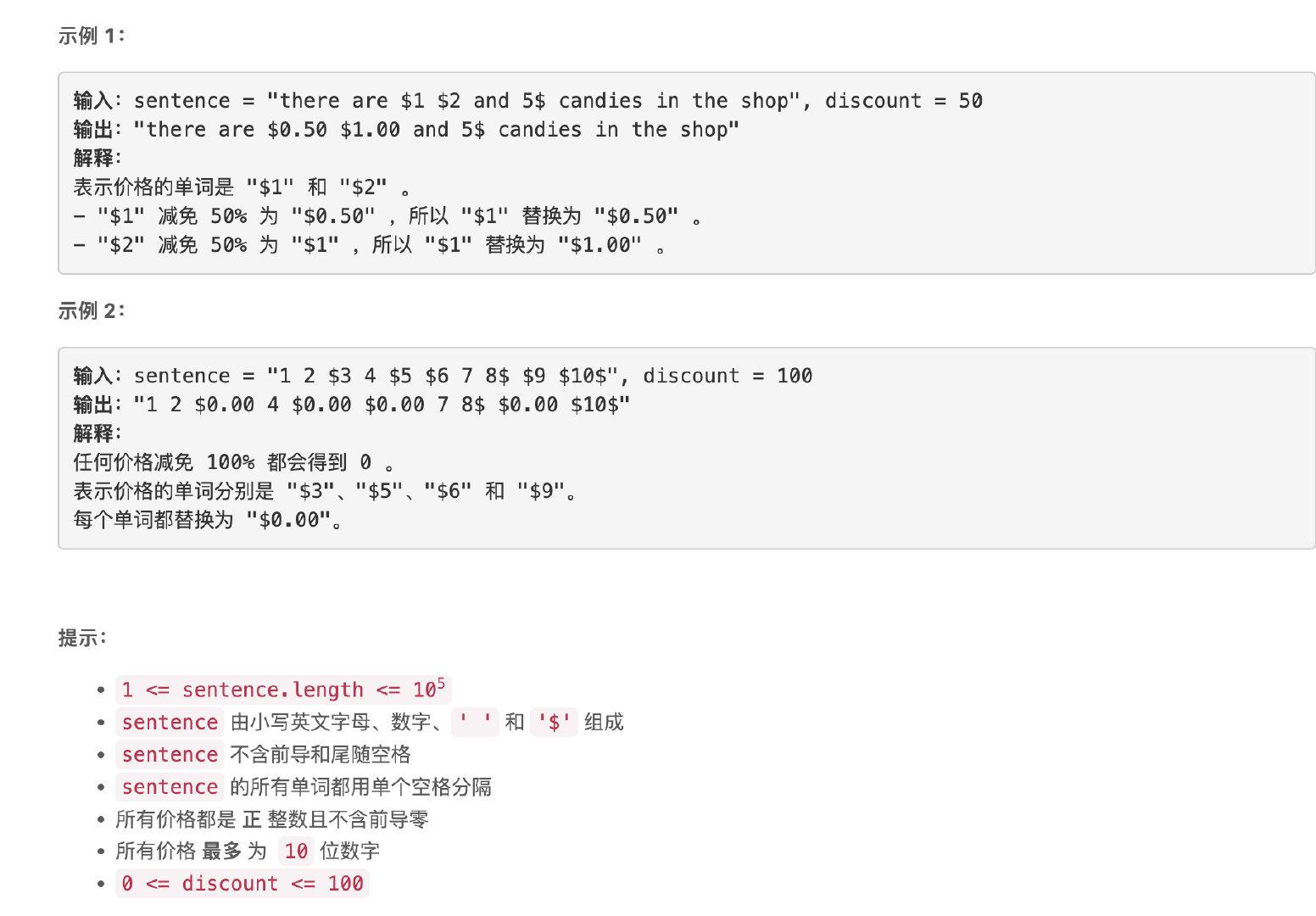### 题解

```cpp string sentence; stringstream ss(sentence); string s;

while (ss >> s) { // do something } ```

```python class Solution: def discountPrices(self, sentence: str, discount: int) -> str: words = sentence.split(' ')

``````    dis = 1.0 - discount / 100

wds = []
for w in words:
if w == '\$':
try:
v = float(w[1:])
w = '\${:.2f}'.format(v * dis)
except:
pass
wds.append(w)

return ' '.join(wds)
``````

```

## 使数组按非递减顺序排列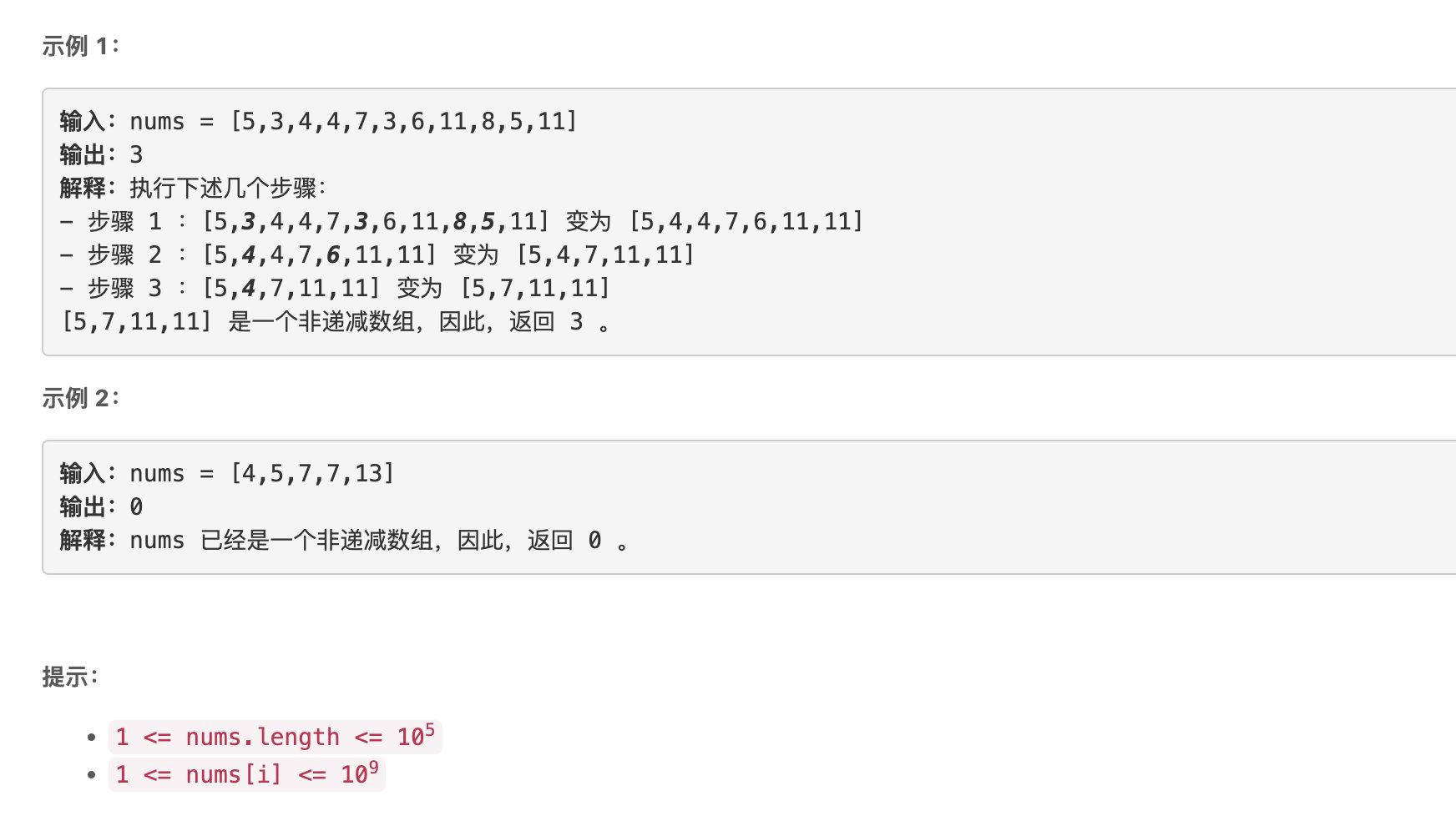### 题解

```cpp class Solution { public: int totalSteps(vector& nums) { list lst(nums.begin(), nums.end());

``````    vector<list<int>::iterator> to_delete;

for (auto p = lst.begin(); next(p) != lst.end(); p++) {
if (*p > *(next(p)))    to_delete.push_back(next(p));
}

int ret = 0;

while (!to_delete.empty()) {
ret++;
vector<list<int>::iterator> buff;

for (auto p: to_delete) {
auto pre = prev(p);
lst.erase(p);
// 判断能否连续删除
if (buff.empty() || buff.back() != pre) {
buff.push_back(pre);
}
}
to_delete.clear();
for (auto p: buff) {
if (next(p) != lst.end() && *p > *(next(p))) to_delete.push_back(next(p));
}
}
return ret;
}
``````

}; ```

## 到达角落需要移除障碍物的最小数目

• 0 表示一个 单元格，
• 1 表示一个可以移除的 障碍物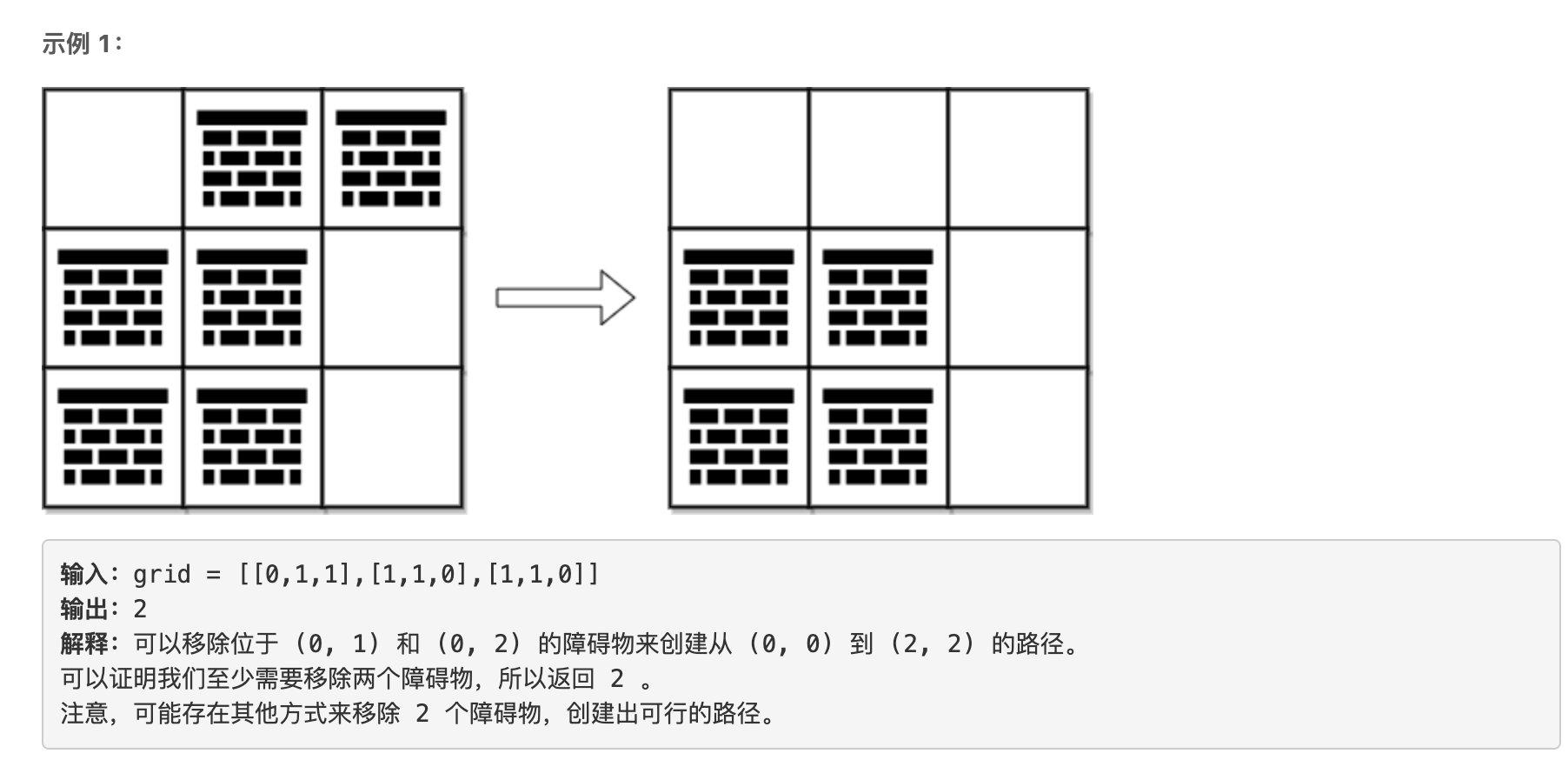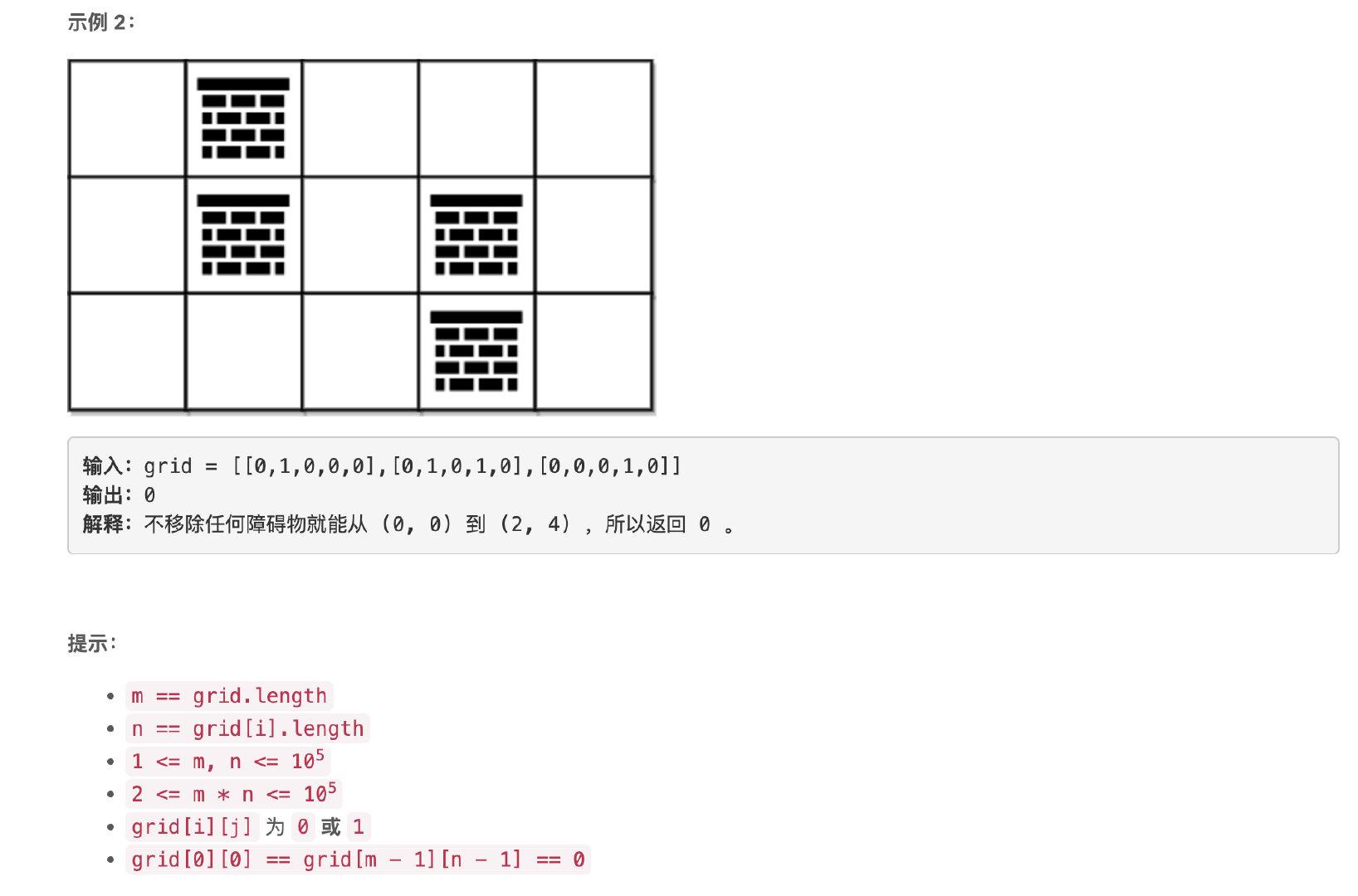### 题解

```cpp typedef pair pii;

class Solution { public: int minimumObstacles(vector>& grid) { int n = grid.size(); int m = grid.size(); vector> dis(n, vector(m, INT_MAX));

``````    queue<pii> cur, nxt;
cur.emplace(0, 0);
dis = 0;

int fx = {{0, 1}, {1, 0}, {-1, 0}, {0, -1}};

int cost = 0;
while (true) {
if (cur.empty()) {
cur = nxt;
nxt = queue<pii>();
cost++;
}
auto [x, y] = cur.front();
cur.pop();
if (x == n-1 && y == m-1) break;

for (int i = 0; i < 4; i++) {
int xx = x + fx[i];
int yy = y + fx[i];
if (xx < 0 || xx >= n || yy < 0 || yy >= m) {
continue;
}
if (grid[xx][yy]) {
if (dis[xx][yy] == INT_MAX) {
nxt.emplace(xx, yy);
dis[xx][yy] = dis[x][y] + 1;
}
}else {
if (dis[xx][yy] == INT_MAX) {
dis[xx][yy] = dis[x][y];
cur.emplace(xx, yy);
}
}
}
}

return cost;
}
``````

}; ```

```cpp typedef pair pii;

class Solution { public: int minimumObstacles(vector>& grid) { int n = grid.size(); int m = grid.size(); vector> dis(n, vector(m, INT_MAX));

``````    deque<pii> que;
que.emplace_front(0, 0);
dis = 0;

int fx = {{0, 1}, {1, 0}, {-1, 0}, {0, -1}};

while (!que.empty()) {
auto [x, y] = que.front();
que.pop_front();

for (int i = 0; i < 4; i++) {
int xx = x + fx[i];
int yy = y + fx[i];
if (xx < 0 || xx >= n || yy < 0 || yy >= m || dis[xx][yy] < INT_MAX) {
continue;
}
dis[xx][yy] = dis[x][y] + grid[xx][yy];
if (!grid[xx][yy]) {
que.emplace_front(xx, yy);
}else {
que.emplace_back(xx, yy);
}
}
}

return dis[n-1][m-1];
}
``````

}; ```